客服热线：133-4342-757518205417169•••# 济南试验机厂家 液压试验机600KN 试验机，材料试验机，拉伸试验机，电液伺服试验机，螺栓拉力机

• 面议

CJK-1478

18205417169 053187062528
• 发货地  山东
• 供货总量  5 台

• 暂无产品目录
 有效期至长期有效 更新时间2020-07-11 04:56 访问次数563

r
r

r

r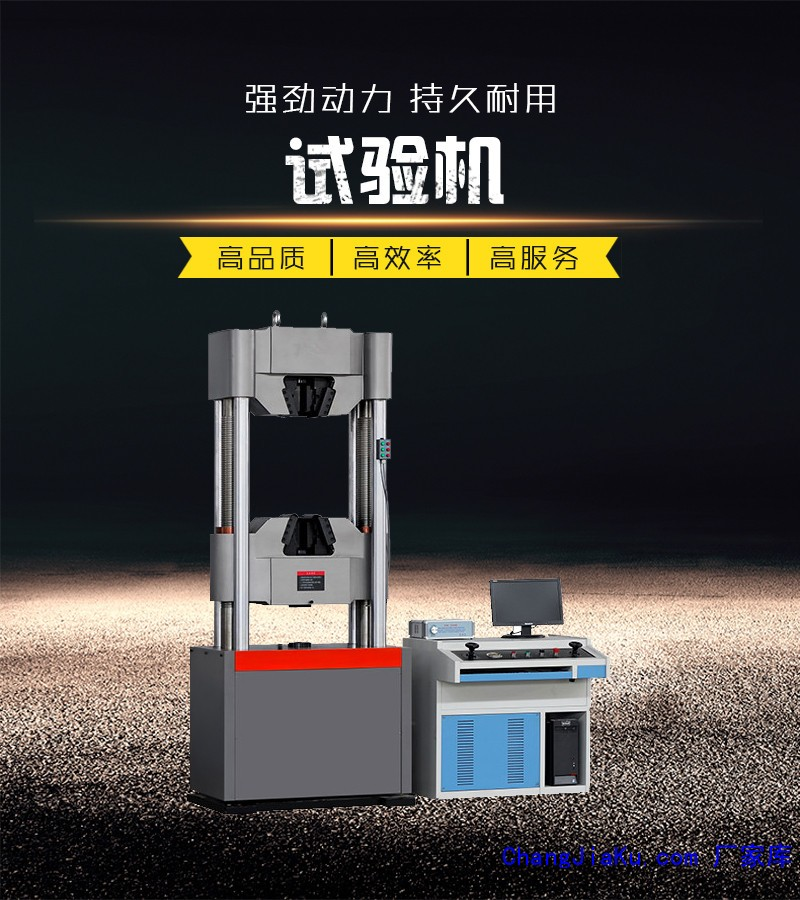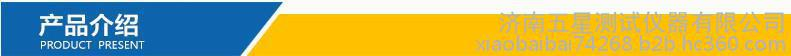r

r

r
r

r

r       本试验机可广泛适用于金属材料的拉伸、压缩、弯曲、剪切试验、广泛应用于大学、科研院所、检测机构、航空航天、军工、冶金、机械制造、交通建设、建工建材等各行业进行精密性的材料研究、材料分析、材料开发和质量控制。r

r

r
r

r

r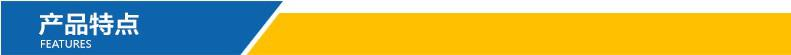r

r

r
r

r

r 1该机型为市场主流机型、高性能、高配置、主机外观精美、空间采用四立柱双丝杠结构、油缸下置式结构，符合人体工程学原理；r

r

r 2主机采用静压油膜间隙密封技术、进口高压齿轮泵和传感器、加载试验过程平稳无冲击，噪声低，试验力值精度高r

r

r 3油源系统配置进口伺服阀，控制精度高；r

r

r 4采集系统由4路精准A/D转换分辨率高达1/300000，全程不分档r

r

r 5软件可实现试验力、位移、变形的三闭环高精度控制，控制方式间能任意组合、平滑切换，完全满足GB/T228-2010（金属材料室温拉伸试验方法）的要求r

r

r 6实时记录、动态显示试验曲线r

r

r 7该试验机控制系统具有网络接口，方便实验室联网操作及试验结果的网络传输r

r

r 8具有试验力过载、超设定、断电、活塞到达极限位置等保护功能。r

r

r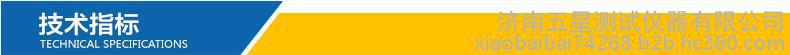r

rr r r r r r r r r r r r r r r r r r r r r r r r r r r r r r r r r r r r r r r r r r r r r r r r r r r r r r r r r r r r r r r r r r r r r r r r r r r r r r r r r r r r r r r r r r r r r r r r r r r r r r r r r r r r r r r r r r r r r r r r r r r r r r r r r
 r r r r r r 规格型号 r r r r r r r WAW-300E r r r r r r r r WAW-600E r r r r r r r r WAW-1000E r r r r r r r r WAW-2000E r r r r 最大试验力 r r r r 300 r r r r 600 r r r r 1000 r r r r 2000 r r r r 试验力测量范围 r r r r 1%-100% r r r r 1%-100% r r r r 1%-100% r r r r 1%-100% r r r r 试验力精度 r r r r ±0.5% r r r r ±0.5% r r r r ±0.5% r r r r ±0.5% r r r r 应力速率控制范围 r r r r 1MP-60MP r r r r 1MP-60MP r r r r 1MP-60MP r r r r 1MP-60MP r r r r 应变速率控制调节 r r r r 0.00025/S-200025/S r r r r 0.00025/S-200025/S r r r r 0.00025/S-200025/S r r r r 0.00025/S-200025/S r r r r 位移速率控制范围 r r r r 0.1／min-50㎜／min r r r r 0.1／min-50㎜／min r r r r 0.1／min-50㎜／min r r r r 0.1／min-50㎜／min r r r r 位移测量精度 r r r r 示值的±0.5%以内 r r r r 示值的±0.5%以内 r r r r 示值的±0.5%以内 r r r r 示值的±0.5%以内 r r r r 变形测量精度 r r r r 示值的±0.5%以内 r r r r 示值的±0.5%以内 r r r r 示值的±0.5%以内 r r r r 示值的±0.5%以内 r r r r 力与变形分辨力 r r r r 最大力与变形量的1 /500000FS r r r r 最大力与变形量的1 /500000FS r r r r 最大力与变形量的1 /500000FS r r r r 最大力与变形量的1 /500000FS r r r r 试样夹持范围 r r r r 圆试样Φ10-Φ32扁试样厚度0-15 r r r r 圆试样Φ10-Φ40扁试样厚度0-30 r r r r 圆试样Φ10-Φ45扁试样厚度0-40 r r r r 圆试样Φ10-Φ70扁试样厚度0-50 r r r r 拉伸最大间距（mm） r r r r 620 r r r r 690 r r r r 780 r r r r 800 r r r r 压缩最大间距（mm） r r r r 550 r r r r 620 r r r r 650 r r r r 700 r r r r 安全保护装置 r r r r 软件保护和机械限位保护 r r r r 软件保护和机械限位保护 r r r r 软件保护和机械限位保护 r r r r 软件保护和机械限位保护 r r r r 超载保护 r r r r 最大力的2%-5% r r r r 最大力的2%-5% r r r r 最大力的2%-5% r r r r 最大力的2%-5% r r r r 电机功率（KW） r r r r 1.5 r r r r 2 r r r r 2 r r r r 2.5 r r r r 主机高度（mm） r r r r 2200 r r r r 2300 r r r r 2500 r r r r 2900 r r r r 主机重量约（KG） r r r r 2200 r r r r 2500 r r r r 3500 r r r r 5500 r r
r

r

r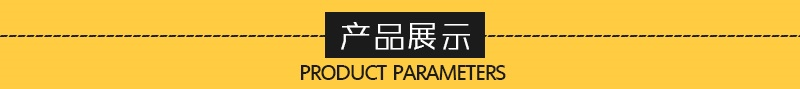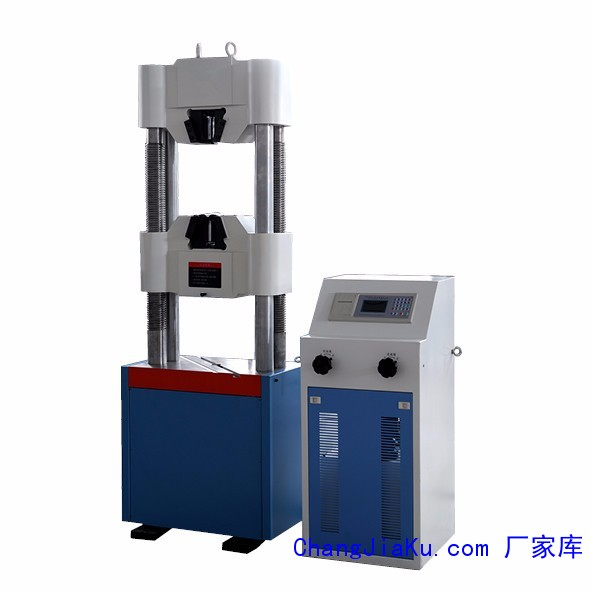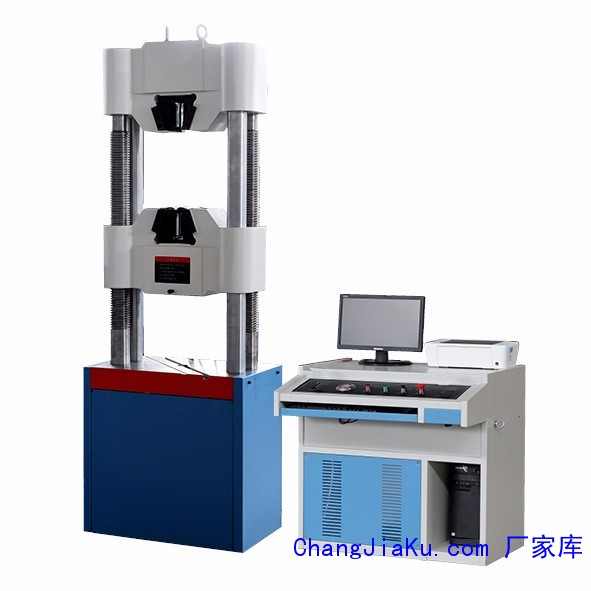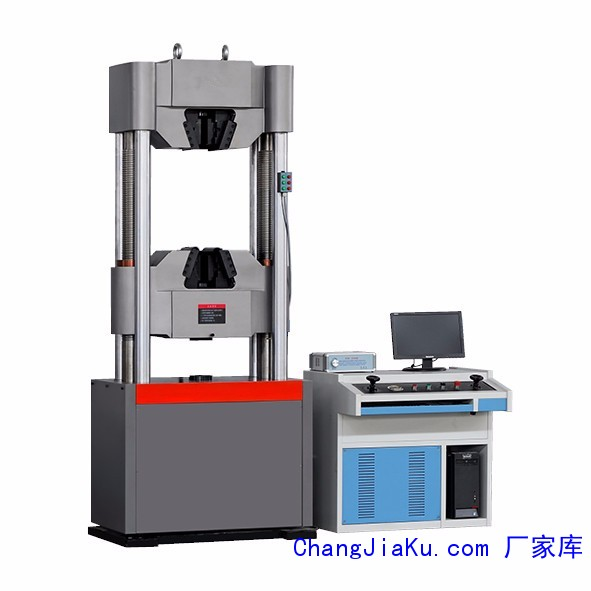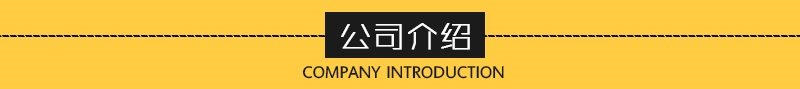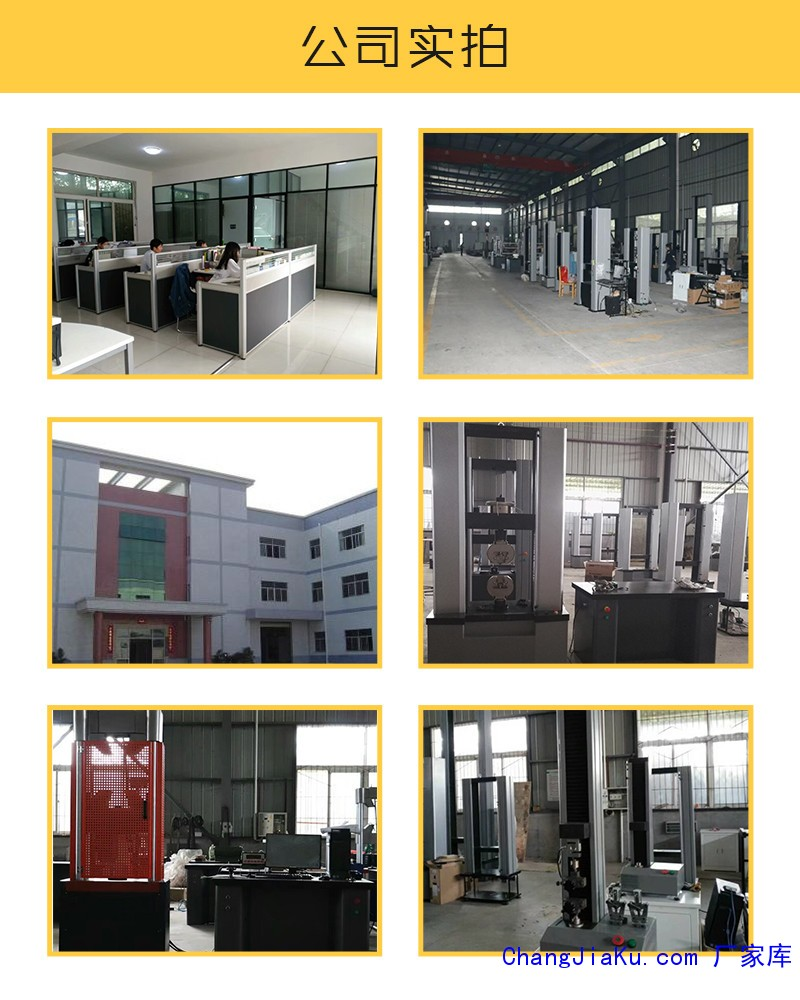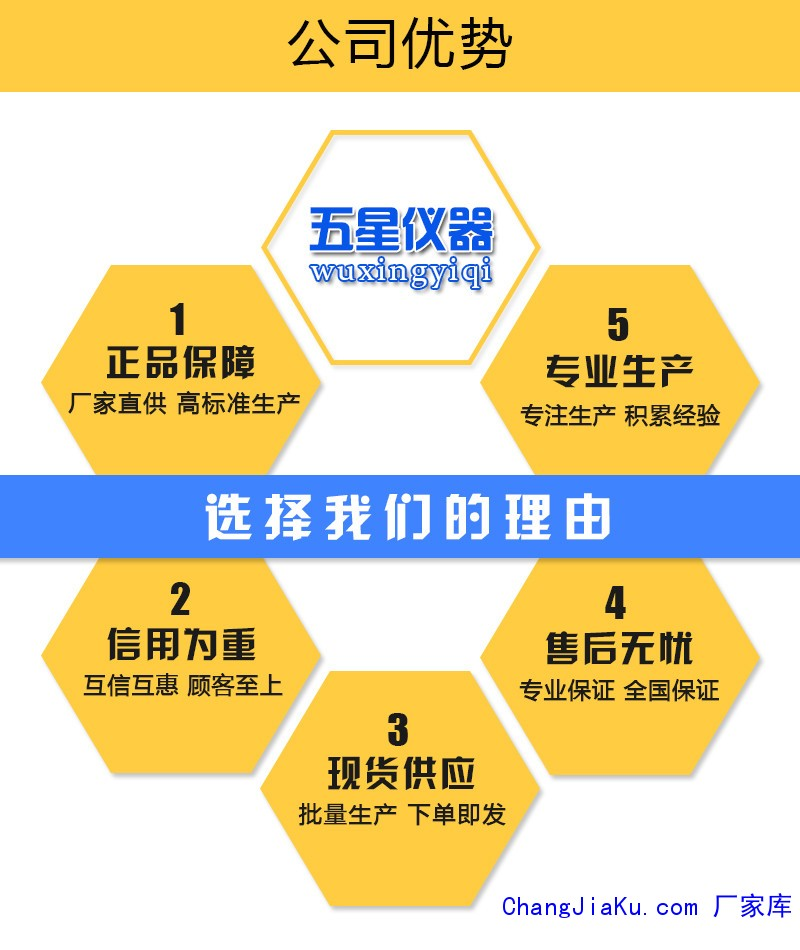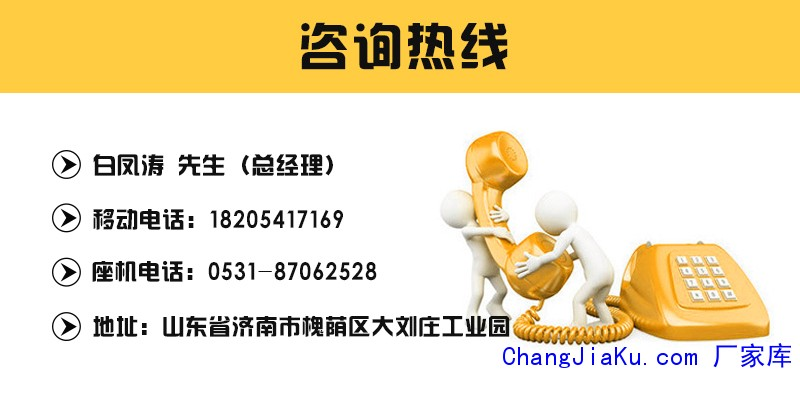r

r r
r r

## 济南五星厂家直销WDW-10D 纸箱压力试验机，rrrrrrr系列包装箱压力试验机主要用于测试包装箱的耐压强度，以防范因纸箱强度不够导致产品在使用、搬运、堆叠、仓储、运输过程中产品变形、损坏等不良现象发生。适用于各类瓦楞纸箱及其他的包装箱抗压强度试验和堆码强度试验。 rrr依据标准： rrr1. **标准GB/T4857.3-92《包运输包装件静载荷堆码试验方法》 rrr2. **标准GB/T4857.4-92《包装运输包装件压力试验方法》 rrr3. ISO2872《包装－完整、满装的运输包装件－压力试验》 rr

## 济南五星 厂家直销JB-B/C 300J 济南冲击机r rrrrrr该试验机是对金属材料在动负荷下抵抗冲击性能进行检测的仪器，能连续和大量地做金属冲击试验，并显示冲击吸收功、冲击韧性、摆锤的旋转角度及打印试验报告等。是金属材料生产厂家、质检部门必备的检测仪器，也是科研单位进行新材料研究不可缺少的测试仪器。该机型可以选配全自动送样系统，可大大的提高试验效率，减轻试验人员劳动强度。 rrrrrr摆锤式冲击试验机执行标准rrr1 GB/T 3808《摆锤式冲击试验机的检验》rrr2 GB/T

## 济南五星厂家直销HGD-100 波纹管压力机 管r rrr软件功能特点r●闭环控制：高性能的调速系统使试验速度实现闭环、自动控制；r●软件系统：采用中文平台下的人机对话操作，操作简单，数据准确；r●自动储存：通过计算机，自动求取**大试验力、断裂力、屈服点、抗拉强度、环刚度、弹性模量、伸长率等参数，并对试验结果自动存储；r●曲线对比：可绘制材料试验的力一伸长、应力一时间、力一时间、伸长一时间等各种特性曲线，并可任选一段进行局部放大、分析；多个式样特性曲线

## 济南五星厂家直销RYQ-3A 软支撑动平衡机，## 济南五星测试试验机厂家 直销 100吨试验机rrrrrrr本试验机可广泛适用于金属材料的拉伸、压缩、弯曲、剪切试验、广泛应用于大学、科研院所、检测机构、航空航天、军工、冶金、机械制造、交通建设、建工建材等各行业进行精密性的材料研究、材料分析、材料开发和质量控制。rrr1该机型为市场主流机型、高性能、高配置、主机外观精美、空间采用四立柱双丝杠结构、油缸下置式结构，符合人体工程学原理；rrr2主机采用静压油膜间隙密封技术、进口高压齿轮泵和传感器、加载试验过程

## 济南五星 WES-1000B材料试验机 数显拉力试r rrr rrrrrr一、性能说明：本试验机为下置式液压万能试验机，主要用于金属材料和水泥、混凝土、塑料等非金属材料的拉伸、压缩、弯曲、剪切等试验。增加附件，可完成钢绞线、钢丝绳、链条、电焊条、胶带及构件的力学性能试验。广泛应用于机械、冶金、交通、建工、建材、大专院校、质量检测等行业和部门。性能可靠，经济实用，是生产和工程中材料检测的理想试验机。 rrr二、产品结构特性 rrr◆主机为两立柱、两丝杠、油缸下置式，拉